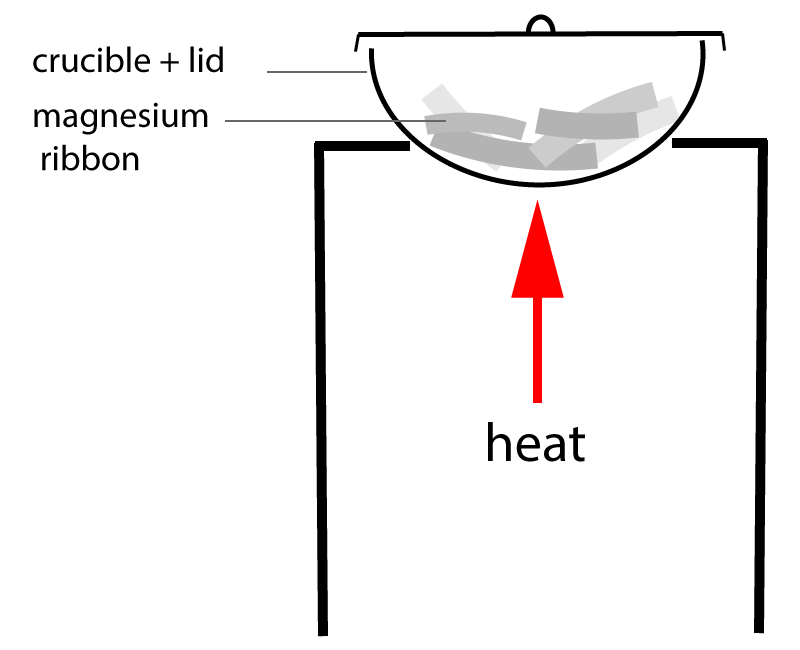10 minutes maximum! Can you do it in 5?

1. The simplest whole number ratio of atoms in a compound is the .
2. The actual number of atoms in a molecule is the .

3. The empirical formula of the compound formed when 0.2 mole of metal X combines with 0.4 mole of non-metal Y is:

• B: XY2
• C: X2Y
• D: X2Y4
4. The simplest formula for the compound formed when 16g of sulfur reacts completely with 24g of oxygen is:
• A:  SO
• B:  SO2
• C:  SO3
• D: S2O3
5. The plastic melamine has the empirical formula CH2N2 and a molar mass of 126g/mol.The molecular formula of melamine is:

• A:  CHN
• B:  CH2N2
• C:  C3H6N6
• D:  C5H10N4

Q6-7. Hydrocarbon X has a relative molecular mass of 42 and contains 85.7% carbon and 14.3% hydrogen.

6. The empirical formula of X is:

• A: CH
• B: CH2
• C: C2H
• D: C6H1
7. The molecular formula of X is:
• A:   CH
• B:   CH2
• C:   C2H4
• D:   C3H6
8. 0.20g of magnesium oxide was formed by heating 0.12g of magnesium ribbon in a crucible.Calculate the number of moles of magnesium and oxygen atoms in the magnesium oxide.

 Moles of magnesium atoms Moles of oxygen atoms A 0.005 0.005 B 0.005 0.0125 C 0.01 0.01 D 0.01 0.025

Q9-10. An oxide of copper was reduced to copper by heating in methane gas in the apparatus shown here:The following results were obtained:

• Mass of ceramic boat = 20.0g
Mass of oxide of copper + ceramic boat (before heating) = 24.0g
Mass of copper + ceramic boat (after heating) = 23.2g

[Relative atomic masses: Cu = 64; O = 16]

9. The masses of copper and oxygen in the original sample are:

 Mass of copper (g) Mass of oxygen (g) A 4.0 4.0 B 4.0 0.8 C 3.2 4.0 D 3.2 0.8
10. The formula of the oxide of copper is:
• A: CuO
• B: CuO4
• C: CuO5
• D: Cu5O4